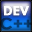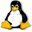Home Latest News Archive Tutorials C++ 2 3 4 Win32 Java OpenGL GLUT OpenGL ES 2 3 4 DirectX VB.NET HTML Photoshop 3dsmax Forums Projects Modelling Links About Us Search Recent Tutorials C / C++ Win32 OpenGL OpenGL ES GLUT## Arithmetic Operators

Tutorials > C++ > Arithmetic Operators

### Introduction

It is almost impossible to create a program that has any use without needing to use some sort of arithmetic. A great deal of mathematics is involved with programming. This tutorial will explain how to accomplish basic arithmetic in C / C++.

Contents of main.cpp :

```#include <iostream>
#include <stdlib.h>

using namespace std;

int main()
{
```

We will be using integers for this tutorial. Many other data types such as floats and doubles may also be used. Even a char has a numerical value known as the ascii value.

```	// Variable Declarations
//-----------------------
int x = 10;
int y = 5;
int z = 0;
```

The basic arithmetic operators can be shown in the following table :

 Operator Description * Multiply / Divide + Plus - Minus

An arithmetic expression can consist of static values as well as variables. You can imagine that the result of the expression replaces it eg.

If x = 5 and y = 10, then z = x + y will evaluate to z = 5 + 10 which will consequently evaluate to z = 15.

I have purposely placed the * and / first as they are in the order of precedence. At school you learnt that multiplication and division should be calculated before addition and subtraction. 5 - 3 * 2 is therefore calculated as 5 - (3 * 2) and NOT as (5 - 3) * 2. You can also place brackets in your expression to explicitly state what operations should occurr when. This is better practice, even if it is not necessary.

```	// Add, Subtract,
// Multiply & Divide
//-------------------
z = x + y;
cout << "10 + 5 = " << z << endl;

z = x - y;
cout << "10 - 5 = " << z << endl;

z = x * y;
cout << "10 + 5 = " << z << endl;

z = x / y;
cout << "10 / 5 = " << z << endl;
```

As the var = var {operator} {expression} is used quite often, other operators are available. Remember that a single number is also an expression in itself. These operators include

*= /= += -=

If you had to explain the operator, you could say operator by eg. multiply by, divide by, increase by, etc. Our example below increases z by 5.

```	z = 5;
z += 5;
cout << "5 + 5 = " << z << endl;
```

Other operator effects are more easily seen be looking at the binary representation of a number. If you do not understand why 22 is equal to 10110, please e-mail me and I'll put up a tutorial on binary numbers. If I do not receive an e-mail, I'll assume that you know how to represent decimal numbers as binary numbers.

```	x = 22; // 10110
y = 17; // 10001
```

Just before we delve into the binary part, another operator MOD can be used. This operator is used by placing a % character. For those who do not know what the MOD operator is, it is essentially the remainder of a division expression.

eg. 10 / 3 = 3 remainder 1

10 / 3 would therefore return 3 and 10 % 3 would return 1.

```	// Mod
//-----
cout << "10 MOD 5 = " << 10 % 5 << endl;
cout << "11 MOD 5 = " << 11 % 5 << endl;
```

I am going to briefly explain the binary operators. Once again, if you are not happy with it, you can e-mail me and I will put up a separate tutorial on binary arithmetic.

The & operator is known as the Bitwise AND operator. The result is only 1 if both operands are 1.

0 & 0 = 0
0 & 1 = 0
1 & 0 = 0
1 & 1 = 1

Therefore :

 10110 : 22 & 10001 : 17 ------- --- 10000 : 16

```	// Binary AND, OR and XOR
//------------------------
cout << "22 & 17 = " << (x & y) << endl;
```

The | operator is known as the Bitwise OR operator. The result is 0 only if both operands are 0.

0 | 0 = 0
0 | 1 = 1
1 | 0 = 1
1 | 1 = 1

Therefore :

 10110 : 22 | 10001 : 17 ------- --- 10111 : 23

```	cout << "22 | 17 = " << (x | y) << endl;
```

The ^ operator is known as the Bitwise XOR operator. The result is 1 only if the operands are different.

0 ^ 0 = 0
0 ^ 1 = 1
1 ^ 0 = 1
1 ^ 1 = 0

Therefore :

 10110 : 22 ^ 10001 : 17 ------- --- 00111 : 7

```	cout << "22 ^ 17 = " << (x ^ y) << endl;
```

Other operators that we will discuss are the increment (++) and decrement (--) operators.
This operator has the form :

var++ or var--

These operators will add or subtract exactly 1 from the variable. You can also place the operator before the variable instead of afterwards. Placing the operator after the variable will cause the variable to only increment after the current line has been processed.

Our first line therefore increases x to 23 and then prints it out.

Our second line shows us what happens if we place the ++ after the variable. It displays the current value and then increments it. We see the new value by looking at the 3rd line.

```	// Increment Operator
//--------------------
cout << "22 + 1 = " << ++x << endl;
cout << "x = " << x++ << endl;
cout << "x + 1 = " << x << endl;
```

The next code segment shows the power of operator precedence. The 30 / 20 is calculated before the addition of the 5. If you wanted to add 5 to 30 first, you would need to rewrite the line as :

z = ((5 + 30) / 20);

```	z = (5 + 30 / 20);
cout << "5 + 30 / 20 = " << z << endl;
```

You may have noticed from the above code segment that the 30 / 20 produces 1 and not 1.5 as you may suspect. This is because you are operating on 2 integers.

The result of an operation is always converted to the biggest of the two operands. We can therefore solve this problem by using 20.0 instead of 20. This causes 20 to be seen as a double. This allows the operation of the 30 / 20 to be converted to a double, therefore returning the desired result.

```	cout << "5 + 30 / 20.0 = " <<
(5 + 30 / 20.0) << endl;

system("pause");

return 0;
}```

You should now be able to accomplish basic Arithmetic in C / C++. I hope you found the tutorial useful as it took quite a while to put it together.

Do not forget about how important brackets are and how operator precedence works. Also remember that the increment and decrement operators can be placed both before and after the variable.

Please let me know of any comments you may have : Contact Me

 Source Files :< Tutorial 10 - Advanced Input Tutorial 12 - Relational Operators >